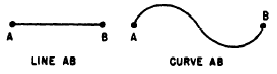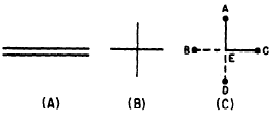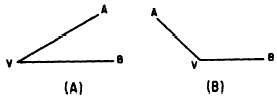Chapter 17 - Plane FiguresCustom SearchCHAPTER 17 PLANE FIGURES The discussion of lines and planes in chapter 1 of this course was limited to their consideration as examples of sets. The present chapter is concerned with lines, angles, and areas as found in various plane (flat) geometric figures. LINES In the strictly mathematical sense, the term "line segment" should be used whenever we refer to the straight line joining some point A to some other point B. However, since the straight lines comprising geometric figures have clearly designated end points, we may simplify our terminology. Throughout the remaining chap used to designate straight line segments, unless stated otherwise. TYPES OF LINES The two basic types of lines in geometry are straight lines and curved lines. A curved -line joining points A a circle, it may be designated as "arc AB."Figure 17-1 -Straight and curved lines.  The term "broken line" in mathematics means a series of two or more straight segments connected end-to-end but not running in the same direction. In mathematics, a series of (See fig. 17-2.)BROKEN LINE DASHED LINE Figure 17-2.-Broken and dashed lines. ORIENTATION Straight lines may be their orientation to the observer’s horizon or in terms of their orientation to each other. For example, lines in the same plane which run beside each other without meeting at any point, no matter how far they are extended, are PARALLEL. (See fig. 17-3 (A).) Lines in the same plane which are not parallel are OBLIQUE. Oblique lines DEB, and BEA) are formed.Figure 17-3.-(A) Parallel lines; (B) and (C) perpendicular lines. Lines parallel to the horizon are HORIZONTAL. Lines perpendicular to the horizon are VERTICAL. ANGLES Lines which meet or cross each other are said to INTERSECT. Angles are formed when angle.Figure 17-4.-(A) Acute angle; (B) obtuse angle. CLASSIFICATION BY SIZE When the sides of an angle are perpendicular to each other, the angle is a RIGHT angle. This the other side is erect or upright. The size of an angle refers to the amount of separation between its sides, and the unit of angular size is the angular DEGREE. A right angle contains 90 degrees, abbreviated 90° . An angle smaller than a right angle is acute; an angle larger than a right angle is obtuse. There 180°. If side AV in figure 17-5 (A) is moved downward, the size of the obtuse angle AVB is in straight line.Integrated Publishing, Inc. - A (SDVOSB) Service Disabled Veteran Owned Small Business